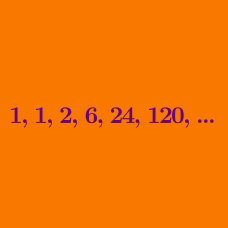Number Theory

# Factorials: Level 3 Challenges

How many of the following 99 integers are primes?

$\begin{array}{c}&100!+2, &100!+3, &100!+4, &\ldots, &100!+100\end{array}$

How many positive integers are divisors of $21!$ but are not divisors of $20!$?

Find the remainder when $70!$ is divided by $5183$.

Note: Don't use a computational device!

$\large \lim_{n \rightarrow \infty} { 2n \choose n } ^ { \frac{1}{n} } = \ ?$

How many trailing zeroes are in the decimal representation of $n=1+\displaystyle{\sum_{k=1}^{2013}k!(k^3+2k^2+3k+1)}?$

×